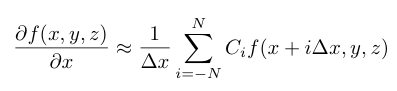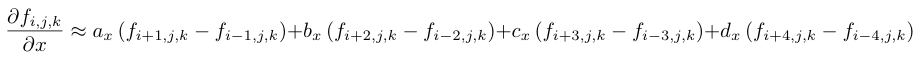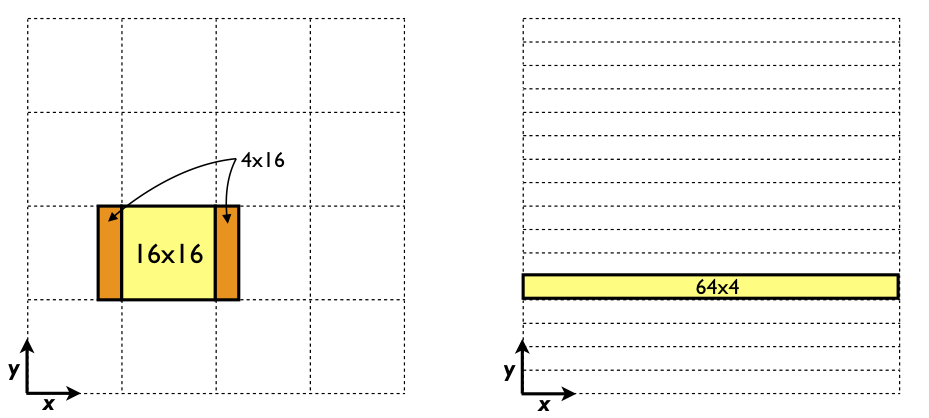# Finite Difference Methods in CUDA Fortran, Part 1CUDA Fortran for Scientists and Engineers shows how high-performance application developers can leverage the power of GPUs using Fortran.

In the last CUDA Fortran post we investigated how shared memory can be used to optimize a matrix transpose, achieving roughly an order of magnitude improvement in effective bandwidth by using shared memory to coalesce global memory access. The topic of today’s post is to show how to use shared memory to enhance data reuse in a finite difference code. In addition to shared memory, we will also discuss constant memory, which is a read-only memory that is cached on chip and is optimized for uniform access across threads in a block (or warp).

# Problem Statement: 3D Finite Difference

Our example uses a three-dimensional grid of size 643. For simplicity we assume periodic boundary conditions and only consider first-order derivatives, although extending the code to calculate higher-order derivatives with other types of boundary conditions is straightforward.

The finite difference method essentially uses a weighted summation of function values at neighboring points to approximate the derivative at a particular point. For a (2N+1)-point stencil with uniform spacing ∆x in the x-direction, the following equation gives a central finite difference scheme for the derivative in x. Equations for the other directions are similar.The coefficients Ci are typically generated from Taylor series expansions and can be chosen to obtain a scheme with desired characteristics such as accuracy, and in the context of partial differential equations, dispersion and dissipation. For explicit finite difference schemes such as the type above, larger stencils typically have a higher order of accuracy. For this post we use a nine-point stencil which has eighth-order accuracy. We also choose a symmetric stencil, shown in the following equation.Here we specify values of the function on the computational grid using the grid indices i, j, k rather than the physical coordinates x, y, z. Here the coefficients are ax = 4/(5 ∆x) , bx = −1/(5 ∆x) , cx = 4/(105 ∆x), and dx = − 1/(280 ∆x), which is a typical eighth-order scheme. For derivatives in the y– and z-directions, the index offsets in the above equation are simply applied to the j and k indices and the coefficients are the same except that ∆y and ∆z are used in place of ∆x.

Because we calculate an approximation to the derivative at each point on the 643 periodic grid, the value of f at each point is used eight times: once for each right-hand-side term in the above expression. In designing a derivative kernel, our goal is to exploit this data reuse by storing blocks of data in shared memory to ensure we fetch the values of f from global memory as few times as possible.

# Data Reuse and Shared Memory

We employ a tiling approach in which each thread block loads a tile of data from the multidimensional grid into shared memory, so that each thread in the block can access all elements of the shared memory tile as needed. How do we choose the best tile shape and size? Some experimentation is required, but characteristics of the finite difference stencil and grid size provide direction.

When choosing a tile shape for stencil calculations, the tiles typically overlap by half of the stencil size, as depicted on the left in the figure below.A better choice of tile (and thread block) size for our problem and data size is a 64 × 4 tile, as depicted on the right in the figure above. This tile avoids overlap altogether when calculating the x-derivative for our one-dimensional stencil on a grid of 643 since the tile contains all points in the direction of the derivative. A minimal tile would have just one pencil, or one-dimensional array of all points in a direction. This would correspond to thread blocks of only 64 threads, however, so from an occupancy standpoint it is beneficial to use multiple pencils per tile. In our finite difference code, available for download on Github, we parameterize the number of pencils to allow experimentation. In addition to loading each value of f only once, every warp of threads loads contiguous data from global memory using this tile and therefore achieves perfectly coalesced accesses to global memory.

# X-Derivative Implementation

The implementation for the x-derivative kernel is:

```attributes(global) subroutine derivative_x(f, df)
implicit none
real, intent(in) :: f(mx,my,mz)
real, intent(out) :: df(mx,my,mz)
real, shared :: f_s(-3:mx+4,sPencils)
integer :: i,j,k,j_l

! j_l is local variant of j for accessing shared memory
k = blockIdx%y

f_s(i,j_l) = f(i,j,k)

! fill in periodic images in shared memory array
if (i <= 4) then
f_s(i-4, j_l) = f_s(mx+i-5,j_l)
f_s(mx+i,j_l) = f_s(i+1, j_l)
endif

df(i,j,k) = &
(ax_c *( f_s(i+1,j_l) - f_s(i-1,j_l) ) &
+bx_c *( f_s(i+2,j_l) - f_s(i-2,j_l) ) &
+cx_c *( f_s(i+3,j_l) - f_s(i-3,j_l) ) &
+dx_c *( f_s(i+4,j_l) - f_s(i-4,j_l) ))

end subroutine derivative_x```

Here `mx`, `my`, and `mz` are the array dimensions which are set to 64, and `sPencils` is 4, which is the number of pencils used to make the shared memory tile. The indices `i`, `j`, and `k` correspond to the coordinates in the 643 mesh. The index `i` can also be used for the x-coordinate in the shared memory tile, while the index `j_l` is the local coordinate in the y-direction for the shared memory tile. This kernel is launched with a block of 64 × `sPencils `threads which calculated the derivatives on a `x` × `y` tile of 64 × `sPencils`, so each thread calculates the derivative at one point.

The shared memory tile is declared with a padding of 4 elements at each end of first index to accommodate the periodic images needed to calculate the derivative at the endpoints of the x-direction.

`  real, shared :: f_s(-3:mx+4,sPencils)`

Data from global memory are read into the shared memory tile for f_s(1:mx,1:sPencils) by the line:

`  f_s(i,j_l) = f(i,j,k)`

These reads from global memory are perfectly coalesced. Data are copied within shared memory to fill out the periodic images in the x-direction by the following code.

```  ! fill in periodic images in shared memory array
if (i <= 4) then
f_s(i-4, j_l) = f_s(mx+i-5,j_l)
f_s(mx+i,j_l) = f_s(i+1, j_l)
endif```

Note that in this operation we are reading from shared memory, not from global memory. Each element is read from global memory only once by the previous statement. Since a thread reads data from shared memory that another thread wrote, we need a `syncthreads()` call before the periodic images are written. Likewise, we need a `syncthreads()` call after the periodic images are written since they are accessed by different threads. After the second `syncthreads()` call, our finite difference approximation is calculated using the following code.

```  df(i,j,k) = &
(ax_c *( f_s(i+1,j_l) - f_s(i-1,j_l) ) &
+bx_c *( f_s(i+2,j_l) - f_s(i-2,j_l) ) &
+cx_c *( f_s(i+3,j_l) - f_s(i-3,j_l) ) &
+dx_c *( f_s(i+4,j_l) - f_s(i-4,j_l) ))```

The coefficients `ax_c`, `bx_c`, `cx_c`, and `dx_c` are declared external to this kernel in constant memory.

## Constant memory

Constant memory is a special-purpose memory space that is read-only from device code, but can be read and written by the host. Constant memory resides in device DRAM, and is cached on-chip.  The constant memory cache has only one read port, but can broadcast data from this port across a warp. This means that constant memory access is effective when all threads in a warp read the same address, but when threads in a warp read different addresses the reads are serialized. Constant memory is perfect for coefficients and other data that are used uniformly across threads, as is the case with our coefficients `ax_c`, `bx_c`, etc.

In CUDA Fortran, constant data must be declared in the declaration section of a module, i.e. before the `contains`, and can be used in any code in the module or any host code that includes the module. In our finite difference code we have the following declaration which uses the `constant` variable attribute.

`real, constant :: ax_c, bx_c, cx_c, dx_c`

Constant data can be initialized by the host using an assignment statement, just as device memory is initialized. Constant memory is used in device code the same way global memory is used. In fact, the only difference between implementing constant and global memory is that constant memory must be declared at module scope, and is declared with the `constant` attribute rather than the `device` attribute. This makes experimenting with constant memory simple, just declare the variable in the module and change the variable attribute.

The x-derivative kernel on a Tesla C2050 achieves the following performance.

```Using shared memory tile of x-y: 64x4
RMS error: 5.7695847E-06
MAX error: 2.3365021E-05
Average time (ms): 3.3689599E-02
Average Bandwidth (GB/s): 57.97412```

We will use this performance as a basis for comparison for derivatives in the other directions, which we will cover in the next CUDA Fortran post.